﻿ 宇宙质量缺失的一种理论猜想 A Theoretical Conjecture about the Lack of Mass in the Universe

Modern Physics
Vol. 09  No. 06 ( 2019 ), Article ID: 33038 , 11 pages
10.12677/MP.2019.96031

A Theoretical Conjecture about the Lack of Mass in the Universe

Xiangyuan Chen

Guangxi University, Nanning Guangxi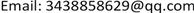Received: Oct. 25th, 2019; accepted: Nov. 13th, 2019; published: Nov. 20th, 2019ABSTRACT

Through the observations of distant celestial bodies, gravitational lens effects, the formation of large-scale structures of the universe, and microwave background radiation, it is shown that if the existing theoretical calculations, galaxies and galaxies in the universe generally have the phenomenon of “loss of quality”. Without the introduction of additional mass, this will not be reasonably explained, and the extra quality introduced is dark matter. In addition to the introduction of dark matter, there is another way to modify the existing gravitational theory. This article does not introduce dark matter. In essence, it does not modify the existing gravitational theory. Instead, it introduces a hypothesis based on observations and tries another novel. We try to make a theoretical explanation for the phenomenon of “loss of quality” that prevails in the universe from another new perspective.

Keywords:Dark Matter, Relativity, Celestial Bodies, Galaxies, Galaxy Clusters1. 引言

1932年，美国加州工学院的瑞士天文学家弗里兹•扎维奇观测漩涡星系旋转速度时，发现星系外侧的旋转速度比牛顿万有引力理论预期的快，因此推测必有数量庞大的质量拉住星系外侧组成，以使其不致因过大的离心力而脱离星系。这个庞大的质量就是后来科学家命名出来的暗物质。后面经过大量的天文观测结果表明，宇宙中所观测到的星系、星系团普遍存在这种“质量缺失”现象。本文不引入暗物质，本质上也没有对现有引力理论进行修改，而是基于观测结果引入一个假设，尝试以另一种全新的角度，对宇宙中普遍存在的“质量缺失”现象进行一种理论解释。

2. 基于现有引力理论和天文观测结果引入假设

${M}_{1}\left(理论质量\right)>{M}_{2}\left(实际质量\right)$ (1)

1. 修改现有引力理论，使得动力学质量和光度质量大致相等。

2. 星系A中存在看不见的物质质量 ${M}_{3}$，使得 ${M}_{2}+{M}_{3}\approx {M}_{1}$

${M}_{1}\left(理论质量\right)={M}_{1}\left(太阳系外空间得到的质量\right)$ (2)

${M}_{2}\left(实际质量\right)={M}_{2}\left(太阳系内空间得到的质量\right)$ (3)

${M}_{1}\left(太阳系外空间得到的质量\right)>{M}_{2}\left(太阳系内空间得到的质量\right)$ (4)

$M\left(太阳系外空间得到的质量\right)>M\left(太阳系内空间得到的质量\right)$ (5)

1) 理论依据：大量关于现有引力理论的检验表明，现有引力理论是正确的，并且适用于太阳系外更遥远广阔的宇宙空间。

2) 实验依据：“质量缺失”现象在宇宙中被证明普遍存在。并且大量关于暗物质粒子的探测搜寻实验中，目前为止我们并没有直接发现暗物质粒子的存在。

${m}_{b}=k{m}_{a}$ (6)

$m\left(k\right)=k{m}_{0}$ (7)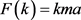(8)

$F\left(k\right)=\frac{G{k}_{1}{k}_{2}mM}{{R}^{2}}$ (9)

$E\left(k\right)=km{c}^{2}$ (10)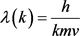(11)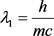(12)

${\lambda }_{2}=\frac{h}{kmc}$ (13)

$\Delta \lambda ={\lambda }_{1}-{\lambda }_{2}=\frac{h\left(k-1\right)}{kmc}$ (14)

$\frac{{t}_{1}}{{t}_{2}}=\frac{{T}_{1}}{{T}_{2}}=\frac{{\lambda }_{1}}{{\lambda }_{2}}=k$ (15)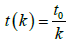(16)

3. 基于假设对宇宙中“质量缺失”现象进行论述

3.1. 宇宙大尺度结构的形成Figure 1. Set of spatial points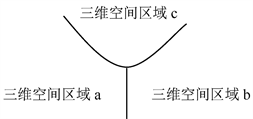Figure 2. Different space regions formed by space expansion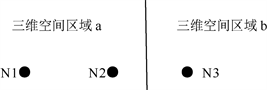Figure 3. Two different spatial regions

1. N2受N1引力为 ${F}_{1}=\frac{G{k}_{1}^{2}{m}_{1}{m}_{2}}{{R}^{2}}$ (17)

2. N2受N3引力为 ${F}_{2}=\frac{G{k}_{1}{k}_{2}{m}_{2}{m}_{3}}{{R}^{2}}$ (18)

1. N2受N1引力加速度 ${a}_{1}=\frac{G{k}_{1}{m}_{1}}{{R}^{2}}$ (19)

2. N2受N3引力加速度 ${a}_{2}=\frac{G{k}_{2}{m}_{3}}{{R}^{2}}$ (20)

$\Delta a={a}_{2}-{a}_{1}=\frac{G{k}_{2}{m}_{3}}{{R}^{2}}-\frac{G{k}_{1}{m}_{1}}{{R}^{2}}=\frac{G\left({k}_{2}{m}_{3}-{k}_{1}{m}_{1}\right)}{{R}^{2}}$ (21)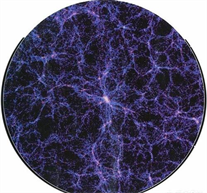Figure 4. Large scale bubble structure simulation of the universe

3.2. 星系、星系团“质量缺失”现象

$V\approx \sqrt{\frac{2GM}{R}}$ (22)

$V\left(k\right)\approx \sqrt{\frac{2kGM}{R}}$ (23)

$V\left(k\right)\approx V\sqrt{k}$ (24)

1. 恒星表面辐射可以近似看做是黑体辐射，根据公式E = hv和公式(11)，在空间相对系数k > 1的空间中，光的频率更大，因此能量更高，恒星辐射能量也更高，因为我们都是在太阳系空间内对遥远天体进行观测，因此我们接收到的天体发出的光的能量实际上变小了。所以对于一个实际光度为L(k)的天体，我们观测到的光度为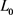，根据光度函数那么可以近似得到：

$L\left(k\right)\approx k{L}_{0}$ (25)

2. 对于理想漩涡星系，星系转动速度可以用氢原子21厘米光谱线的多普勒频率移动效应来测量,其中恒星或者气体轨道运动引起的多普勒频移可以由以下公式近似表示：

$\frac{\Delta \lambda }{\lambda }\approx \frac{{V}_{r}}{C}$ (26)

$V\left(k\right)\approx {V}_{0}\left(\frac{\Delta \lambda -\Delta {\lambda }_{k}}{\Delta \lambda }\right)$ (27)

3. 恒星质量越大一般寿命越短，在天文学上恒星的寿命和质量一般有如下关系

$t\propto \frac{1}{{M}^{a-1}}$ (28)

$t\left(k\right)\propto \frac{k}{{\left(kM\right)}^{a-1}}$ (29)

$\Delta t\propto t-t\left(k\right)\propto \frac{1}{{M}^{a-1}}-\frac{k}{{\left(kM\right)}^{a-1}}\propto \frac{k\left({k}^{\left(a-2\right)}-1\right)}{{\left(kM\right)}^{a-1}}$ (30)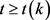(31)

1. 当k > 1时，理论上可以证明在这种空间中星系、星系团会出现“质量缺失”现象。而且k越大“质量缺失”比例越大。

2. 当k ≈ 1时，理论上可以证明在这种空间中星系、星系团“质量缺失”现象不会很明显。

3. 当k < 1时，理论上可以证明在这种空间中星系、星系团会出现和第一种情况相反的情形。

4. 对提出的假设进行初步验证的一种直接方法

$z\equiv \frac{\Delta \lambda }{{\lambda }_{0}}=\frac{1}{c}{H}_{0}d$ (32)

5. 结语

1. 我们取地球作为静止参考系，那么根据狭义相对论，惯性参考系中的运动质量(空间相对系数k = 1的空间中)可以表示为：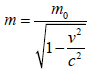2. 我们取地球作为静止参考系，那么根据本文所提出的假设，在一个空间相对系数为k的空间中，根据公式(7)其中的静止质量可以表示为： $m\left(k\right)=k{m}_{0}$(33)

1. 麦克斯韦理论 → 得到光速c结果 → 引入以太解释 → 抛弃以太 → 得到运动质量变化结论。

2. 广义相对论 → 得到“质量缺失”结果 → 引入暗物质进行解释 → 抛弃暗物质→ 得到静止质量变化结论。

A Theoretical Conjecture about the Lack of Mass in the Universe[J]. 现代物理, 2019, 09(06): 332-342. https://doi.org/10.12677/MP.2019.96031

1. 1. Behnke, E., Besnier, M., Bhattacharjee, P., Dai, X., Das, M., Davour, A., Debris, F., Dhungana, N., Farine, J., Fines-Neuschild, M., Gagnebin, S., Giroux, G., Grace, E., Jackson, C.M., Kamaha, A., Krauss, C.B., Lafrenière, M., Laurin, M., Lawson, I., Lessard, L., Levine, I., Marlisov, D., Martin, J.-P., Mitra, P., Noble, A.J., Plante, A., Podviyanuk, R., Pospisil, S., Scallon, O., Seth, S., Starinski, N., Stekl, I., Wichoski, U. and Zacek, V. (2017) Final Results of the PICASSO Dark Matter Search Experiment. Astroparticle Physics, 85-92.
https://doi.org/10.1016/j.astropartphys.2017.02.005

2. 2. Swinbank, M. (2017) Astrophysics: Distant Galaxies Lack Dark Matter. Nature, 543, 318-319.
https://doi.org/10.1038/543318a

3. 3. van Dokkum, P., Danieli, S., Cohen, Y., Merritt, A., Romanowsky, A.J., Abraham, R., Brodie, J., Conroy, C., Lokhorst, D., Mowla, L., O’Sullivan, E. and Zhang, J.L. (2018) A Galaxy Lacking Dark Matter. Nature, 555, 629-632.
https://doi.org/10.1038/nature25767